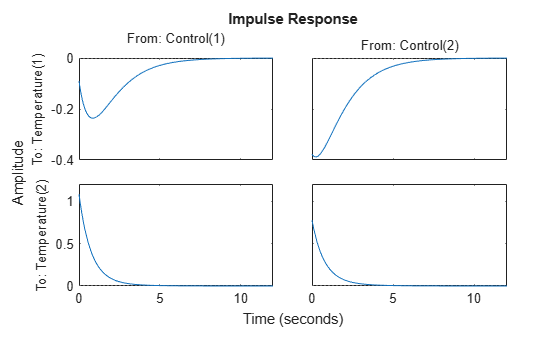# Time-Domain Responses of MIMO Model

This example shows how to obtain impulse response data and plots for a multi-input, multi-output (MIMO) model using `impulse`. You can use the same techniques to obtain other types of time-domain responses of MIMO models.

Create a MIMO model and plot its response to a `t` = 0 impulse at all inputs.

```H = rss(2,2,2); H.InputName = 'Control'; H.OutputName = 'Temperature'; impulse(H)````impulse` plots the response of each output to an impulse applied at each input. (Because `rss` generates a random state-space model, you might see different responses from those pictured.) The first column of plots shows the response of each output to an impulse applied at the first input, `Control(1)`. The second column shows the response of each output to an impulse applied at the second input, `Control(2)`.

Calculate the impulse responses of all channels of `H`, and examine the size of the output.

```[y,t] = impulse(H); size(y)```
```ans = 1×3 207 2 2 ```

The first dimension of the data array `y` is the number of samples in the time vector `t`. The `impulse` command determines this number automatically if you do not supply a time vector. The remaining dimensions of `y` are the numbers of outputs and inputs in `H`. Thus, `y(:,i,j)` is the response at the `i` th output of `H` to an impulse applied at the `j` th input.